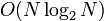# Nondeterministic black-box group algorithm for abelianness testing

## Summary

Item Value
Problem being solved abelianness testing problem: determine whether a given group is abelian.
Input format a finite group$G$ given via an encoding of a group
Output format Yes/No depending on whether$G$ is abelian
In the No case it is also possible to output a pair of elements that do not commute.
Theoretical significance This shows that the abelianness testing problem is$NP \cap coNP$. However, it is already known that the problem is in$P$, so this is not really new information.
Running time$O(N\log_2N)$ times the time taken for group operations.
Competing algorithms black-box group algorithm for abelianness testing: takes time$O(N^2)$ times the time for group operations, but is deterministic.
randomized black-box group algorithm for abelianness testing: takes polylog time (much faster) and is random, but provides proof only for non-abelianness, not for abelianness (i.e., only puts the problem in co-RP and not in RP).